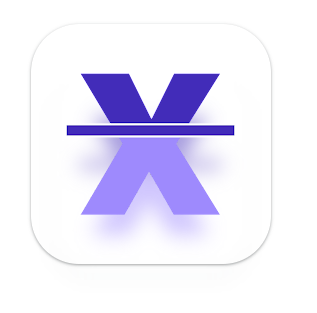# ScanMath – Algebra Solver

by | Sep 26, 2022 | Android App, Learning Apps | 0 comments

Because of technological advancements, smartphones and tablets have apps to solve math problems. These math solution apps make it as simple as clicking a picture to solve complex mathematical problems and provide step-by-step instructions on how it arrived at the answer.

These math solver apps will assist you in tracking formulas and learning algebra by allowing you to solve equations by simply pointing to the camera. Scan Math covers most levels of mathematics, such as trigonometry, statistics, algebra, calculus, arithmetic, and more. They provide online assistance to students of all grades, ages 6 to 18.

If you get stuck on a math problem, scan it with Scan Math to get an instant answer and detailed solution. Scan Math is a must-have App for students of all levels.

## Scan Math Covers The Majority Of Math Subjects:

• Pre-Algebra: Whole numbers, arithmetic, fractions, decimal numbers, integers, roots, factors, percentages, ratios, rates, and proportions are all covered.
• Linear equations and inequalities, quadratic equations, matrices, logarithms, functions, and graphing
• Trigonometric functions, the Pythagorean theorem, identities, conic sections, vectors, matrices, complex numbers, sequences, and series
• Limits, derivatives, and integrals are examples of calculus concepts.
• Statistics: Factorials, combinations
• Languages supported: English, Spanish, French, Portuguese, and Italian.Scan Math is your math assistant app. Scan Math will illustrate the answers and solutions with step-by-step instructions after you snap the math problem.

Understandably, math is one of the most stressful subjects you can face. Whether or not you’re good at it, solving complex math problems during concepts like calculus or trigonometry can be difficult. Even fundamental arithmetic problems can be complicated at times. Fortunately, numerous apps available for your Android phone can assist you in solving math problems.

## Get Better at Mathematics

Because everyone is different, some people may excel at math while others may not. If you struggle with more complicated problems, the ScanMath – Algebra Solver app can assist you with homework and other assignment solutions.